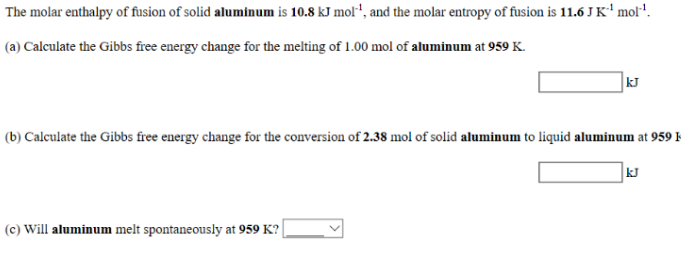# Problem: The molar enthalpy of fusion of solid aluminum is 10.8 kJ mol-1, and the molar entropy of fusion is 11.6 J K-1mol-1. (a) Calculate the Gibbs free energy change for the melting of 1.00 mol of aluminum at 959 K. (b) Calculate the Gibbs free energy change for the conversion of 2.38 mol of solid aluminum to liquid aluminum at 959 K. (c) Will aluminum melt spontaneously at 959 K?

###### FREE Expert Solution
82% (474 ratings)###### Problem Details

The molar enthalpy of fusion of solid aluminum is 10.8 kJ mol-1, and the molar entropy of fusion is 11.6 J K-1mol-1

(a) Calculate the Gibbs free energy change for the melting of 1.00 mol of aluminum at 959 K.

(b) Calculate the Gibbs free energy change for the conversion of 2.38 mol of solid aluminum to liquid aluminum at 959 K.

(c) Will aluminum melt spontaneously at 959 K?